1.800.221.5175
Core Program
Full Access
Core Program
Full Access
High-Impact Tutoring
Core Program
Full Access
National Program
Full Access
New York
Critical Thinking for Active Math Minds
Preparing for Standards Based Assessments
Vocabulary Workshop, Tools for Comprehension Grades 1–5
Print Program
Interactive Edition
Print Program
Interactive Edition
Vocabulary Workshop, Tools for Excellence Grades 6–12+
Print Program
Interactive Edition
Vocabulary for Success
Progress English Language Arts Grades K–8
National Program
Full Access
High-Impact Tutoring
New York
Grammar Workshop, Tools for Writing
Grammar for Writing
Writing a Research Paper
Writing Workshop
Print Program
Interactive Practice Bundle
Fluency Booster Practice Book
Interactive Assessments
High-Impact Tutoring
Building Reading Success with Wiley Blevins
Print Program
Interactive Edition
Professional Learning

## How Math Games and Activities Increase Student Proficiency in Grade 8

Math games and activities can be an excellent tool to supplement and support math instruction. Using math games in your classroom allow students to practice mathematics in fun but also impactful ways. Students love games because they are engaging and exciting, and teachers love games because they help students practice what they've learned.

Students in Grade 8 build upon prior content that helps them to analyze equations, compare functions, analyze 2D and 3D space, and understand quantitative relationships. They solve a variety of problems and know about the Pythagorean Theorem. They understand the slope of a line as a constant rate of change, and how to use a variety of models and appropriate tools strategically.

## Dynamic Model

for Angles of a Transversal

### Use a physical model to show how angles formed by a transversal change along with one another.

1. Cut out 3 strips of cardboard and assemble them with fasteners to form a transversal and two parallel lines.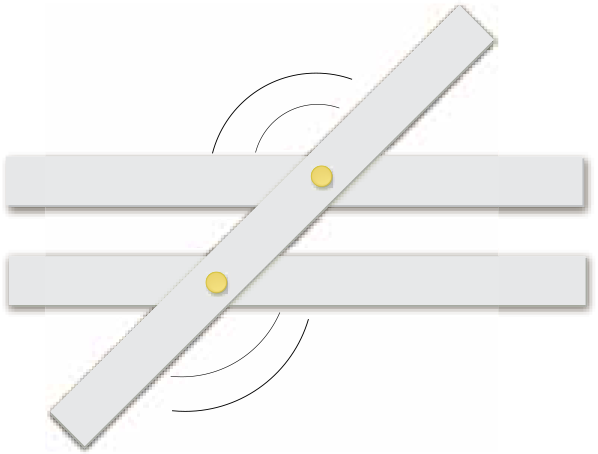1. Rotate the transversal. As the angles change in size, focus students’ attention on pairs of angles that remain congruent and pairs that remain supplementary.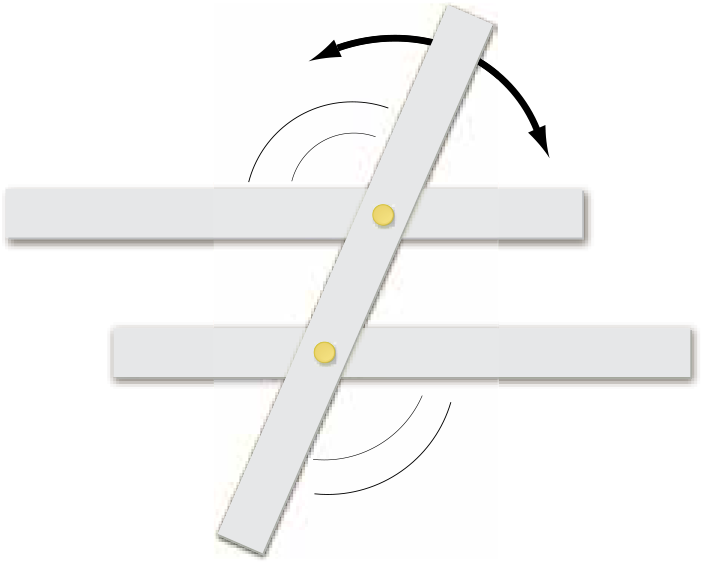1. Add another cardboard strip that will act as a second transversal and that is parallel to the first. Focus students’ attention to the parallelogram that is formed. Point out the congruent angle pairs and how opposite angles within the parallelogram are also congruent.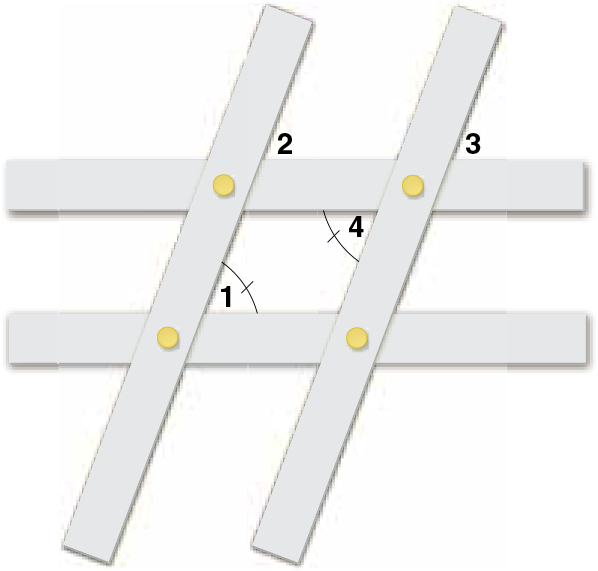#### Inquiry Strategies

1. Analyze Ask, “How do you need to move the transversal so that all eight angles are congruent?” Ask, “Use the congruence of vertical angles and the congruence of corresponding angles to show that opposite angles in a parallelogram are congruent.” Move the transversal so that it is perpendicular to the two parallel strips; Student responses should focus on the principal that angles 2 and 3 correspond to angle 1, while angles 3 and 4 are vertical angles. Therefore, angles 1 and 4 are congruent.
2. Create Have students draw a diagram of a transversal intersecting three parallel lines to form 12 angles. Ask students to label sets of angles that are always congruent. Students should label all the obtuse angles congruent and all the acute angles congruent.

## Visual Model

for Area of a Circle Formula

### Take apart and reconfigure the interior of a circle to show why the area formula A = πr2 makes sense.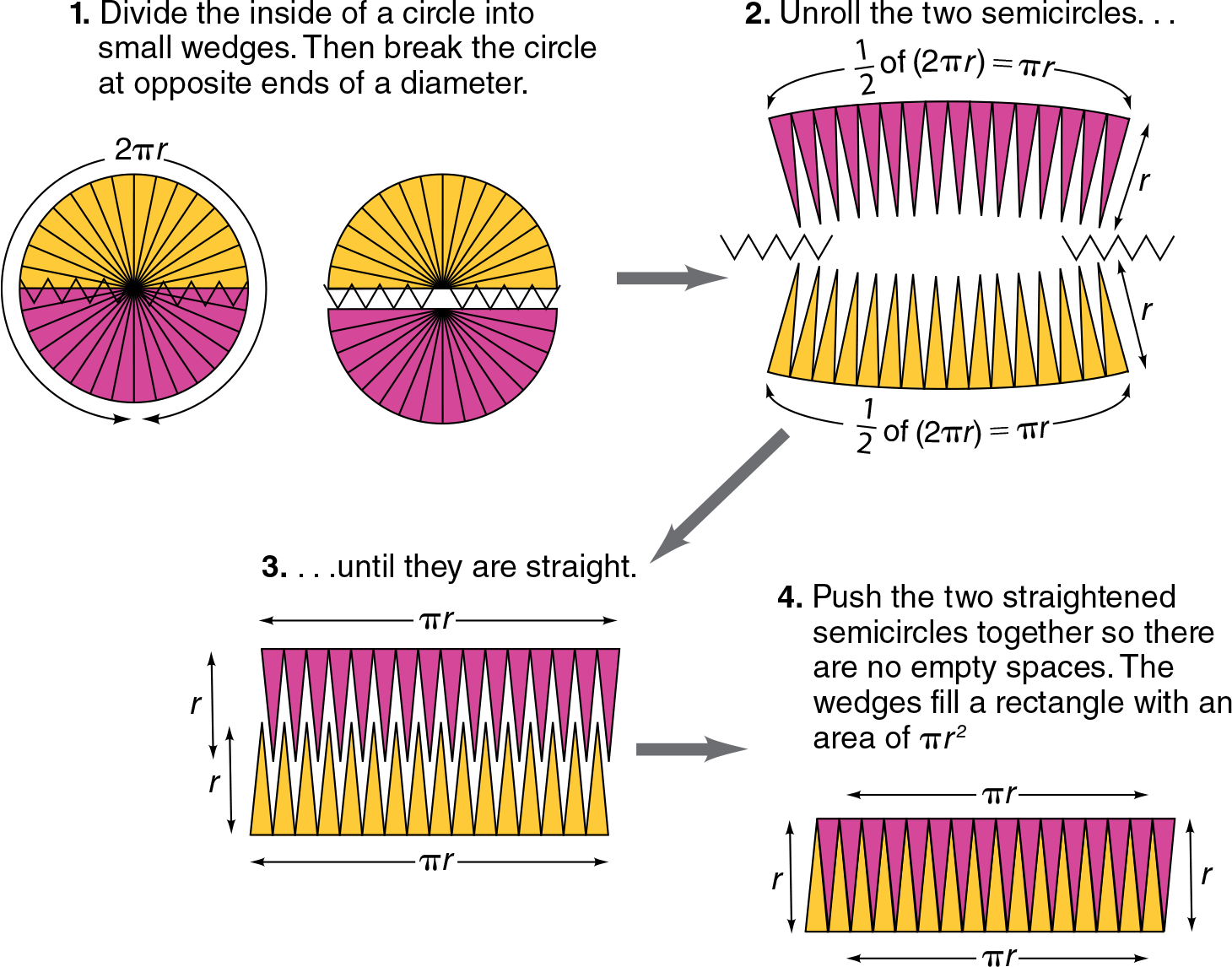#### Inquiry Strategies

1. Understand Ask students to explain why the area of the rectangle in the last step is the same as the area of the circle in the first step. Possible response: No area is added or taken away; the pieces of the circle’s interior have simply been rearranged.
2. Evaluate Have students look at the figure in the last step. Ask them to explain how the figure becomes more and more like a true rectangle as the original circle is divided into smaller and smaller wedges. Possible response: As the wedges decrease in size, their curved ends become shorter, and so the row of ends looks less like a series of curves and more like a line segment.

## Area Model

for Probability

### Use a circle to show why the product 1⁄6 x 1⁄6 can be used to find the probability of rolling two 4s when a 1–6 number cube is rolled twice.

1. Divide a circle into 6 equal sections to show each segment you would expect to appear when you roll a cube.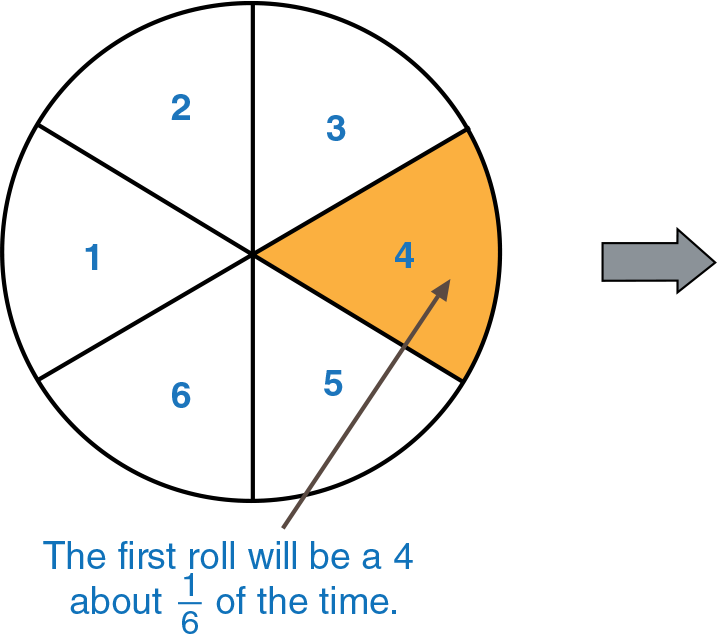1. Divide the “4” section into 6 equal subsections, labeled to show each possible roll 1 through 6. These subsections also show how often a roll of 4 could be followed by another roll of 4.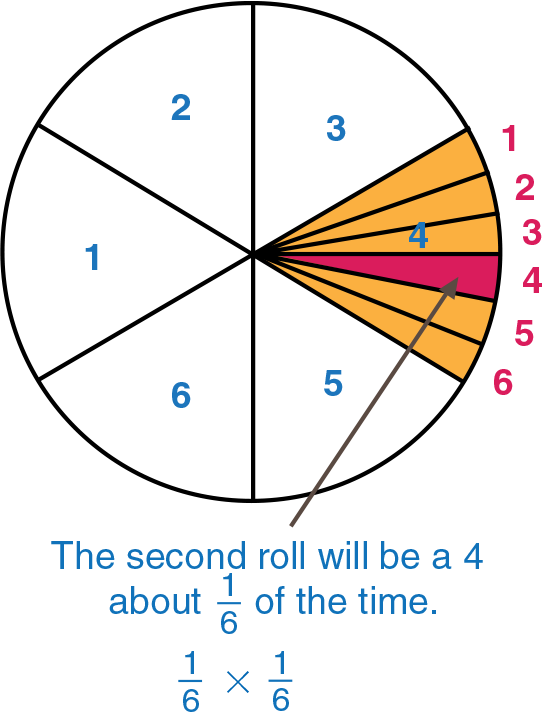#### Inquiry Strategies

1. Understand Ask students to explain different parts of the model. For example, ask, “What does the whole circle at the left represent? Why are the sections equal? What does the whole circle at the right represent? Why are the subsections in the 4 area divided into 6 equal segments?” The total possibilities when the cube is rolled; on any roll each number has an equal chance to come up; the total possibilities when the cube is rolled a second time, when the result of the first roll was a 4; to show the equal possibility of rolling each number on a second roll of the cube
2. Create Have students use a square to make an area model for the probability of tossing three tails when tossing a fair coin three times. Models should look something like those below.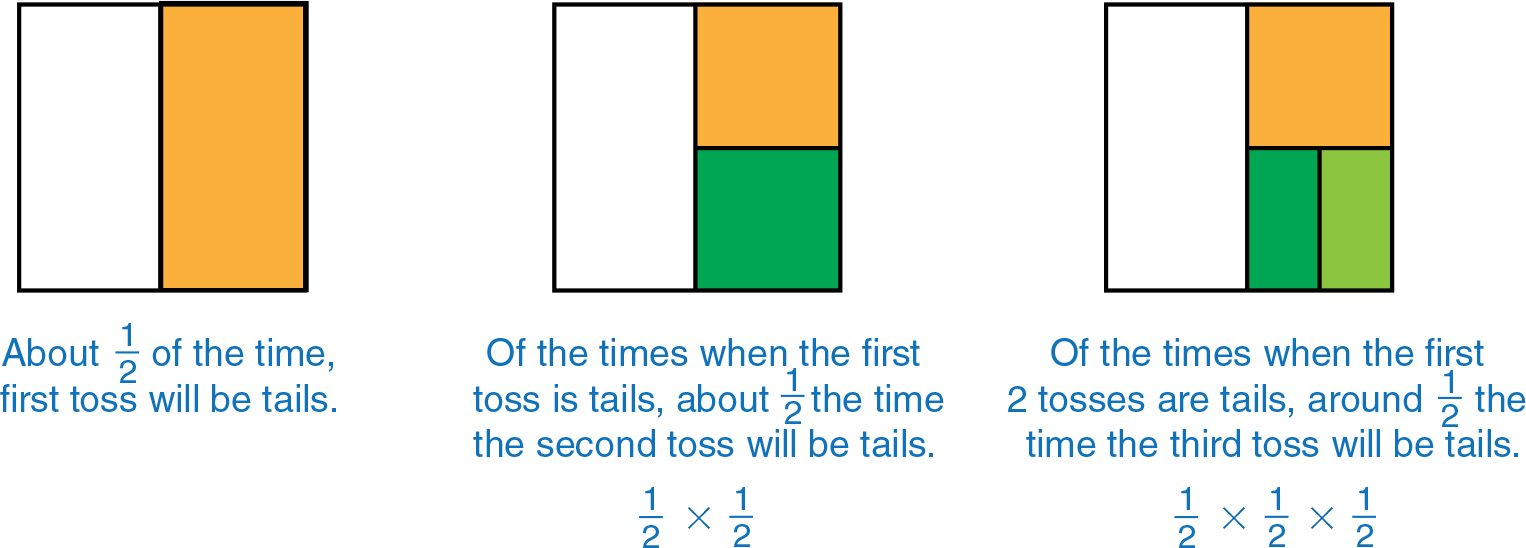## Visual Model

for Distribution Displays

### Display a stem-and-leaf plot, a histogram, and a box-and-whisker plot, all made from the same set of data, such as the monthly temperature recorded twice over the period of one year. Draw arrows to illustrate how the plots use different ways of showing the same data distribution.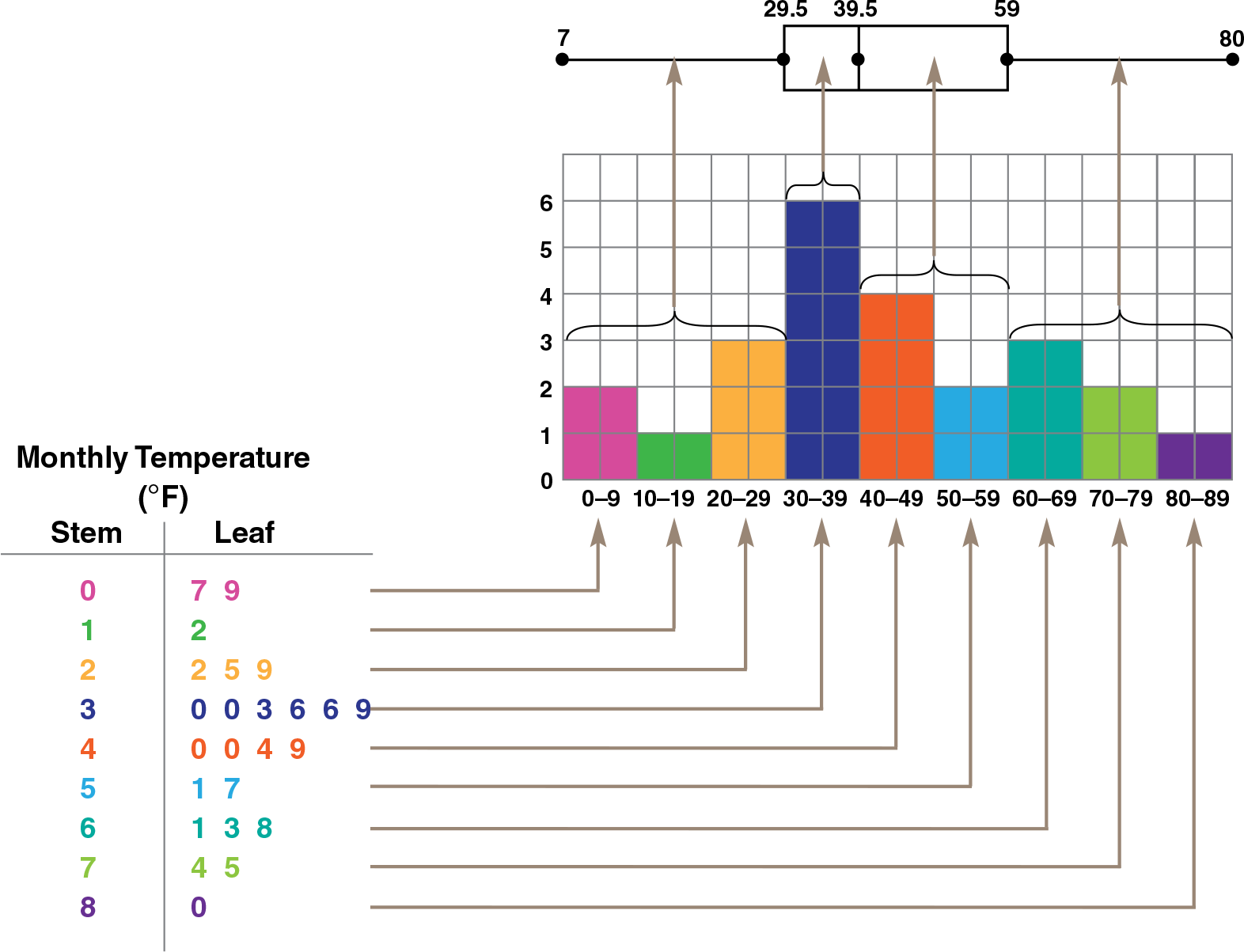#### Inquiry Strategies

1. Analyze Call attention to the 30–39 interval on the stem-and-leaf plot and the histogram. Ask, “Why do the longest line of data on the stem-and-leaf plot and the tallest bar on the histogram correspond to the shortest part of the box-and-whisker plot?” The purple row on the stem-and-leaf plot and the purple bar on the histogram each represent the 6 data values in the 30–39 interval, which represents the highest frequency. Those 6 values represent one fourth of all the values. So this single interval constitutes one fourth of the data. Further, the shortest part of the box-and-whisker plot represents the clustering of data.
2. Apply Have students imagine that the three types of displays above also include 16 more data values, all in the 90–99 interval. Ask, “Which of the displays would require the most work to change? Explain.” Possible response: The box-and-whisker plot, because the other two could be altered just by adding a row or bar. The box-and-whisker plot would require recalculating the quartiles and redrawing the plot. In doing so, the box-and-whisker plot would show new data clusters and ranges.

## Rectangle Model

for Inverse Functions

### Use rectangles of equal area to show the shape of the graph of an inverse function.

1. Write the equation: xy = 60 on the board.
2. Draw a short table of convenient values, where x represents length and y represents width, as shown.
3. Plot (4, 15). Then use that point to outline a rectangle as shown in red. Note that the dimensions are 4 and 15, and that the area is equal to 60.
4. As you plot each point, draw a rectangle and point out that the area is equal to 60.
5. Draw a curve through the points.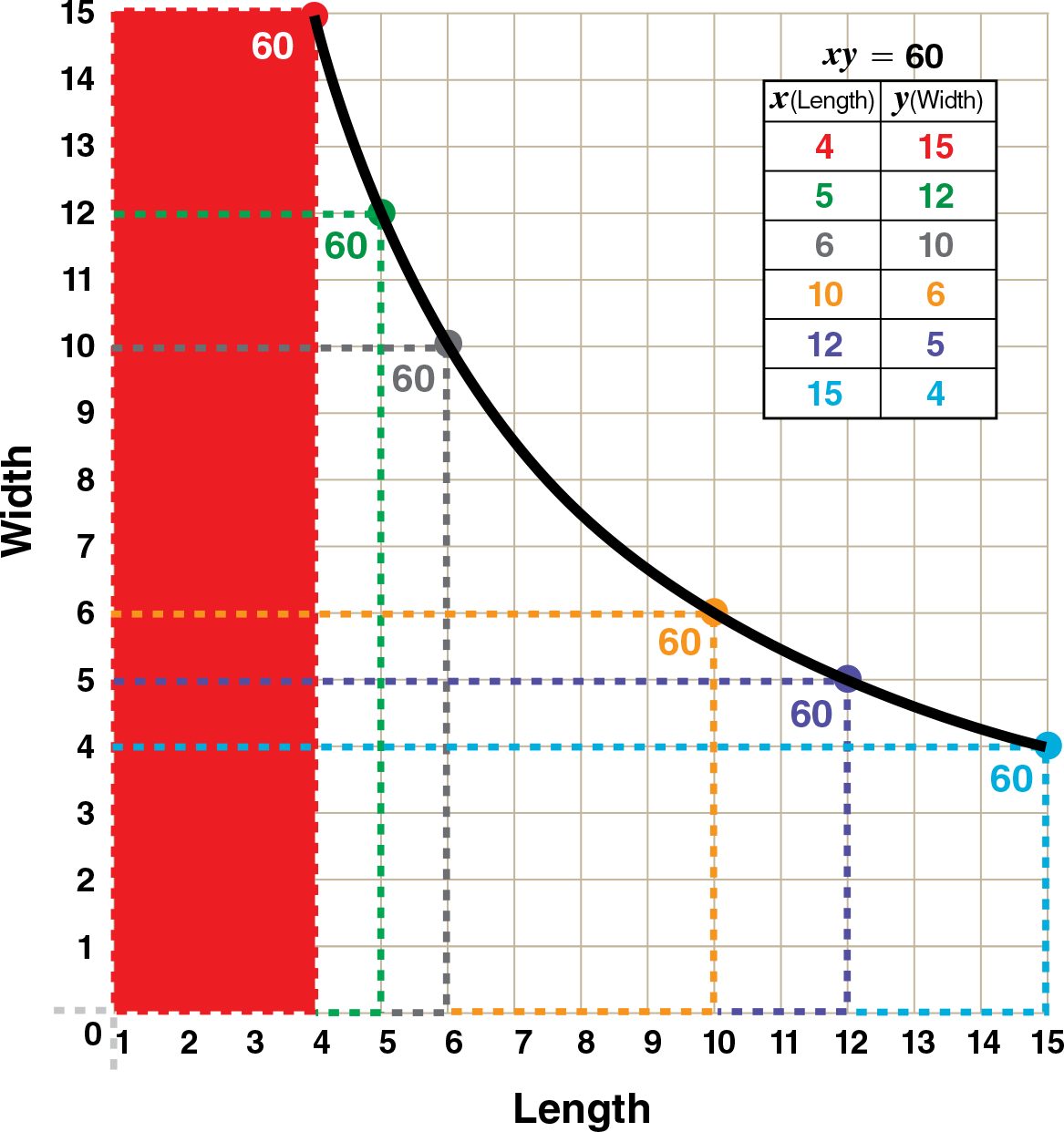#### Inquiry Strategies

1. Understand Ask students to explain how they know that each plotted point on the graph satisfies the equation xy = 60. Possible response: Each x and y value represents the length and width of a rectangle with an area of 60, so their product must equal 60.
2. Analyze Why will the curve never touch the x- or y-axis? Possible response: If the curve intersected the x or y axis, that would mean the value of x or y would be zero. If the length or width were zero, the area could not be 60; there would be no rectangle. Even further, this would contradict the definition of an inverse variation, y = Kx

## Linear Relationship

Distance and Weight

### Using a wooden board and a scale, students will discover the linear relationship between distance and weight. This activity requires a bathroom scale, a strong wooden board, approximately 6 feet long, and several objects to be weighed, such as backpacks. Starting about 6 inches from the edge of the board (to allow overhang on the scale) mark off 12-inch intervals. Place one end of the board on the scale and the other on a textbook of about the same height.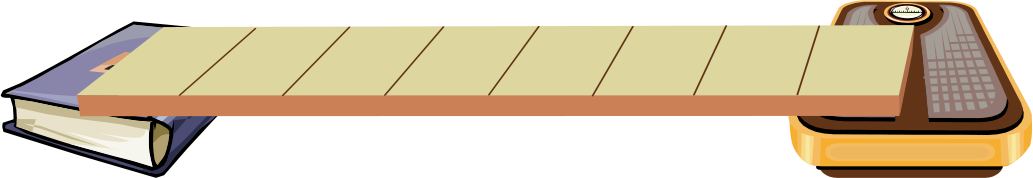Begin by placing an object on the scale, and read aloud the weight shown. Have students record the object and weight in a chart, such as below. Move the object to the 12-inch mark and have students record that weight. Continue moving the object to each successive mark and have students record the weights at each point.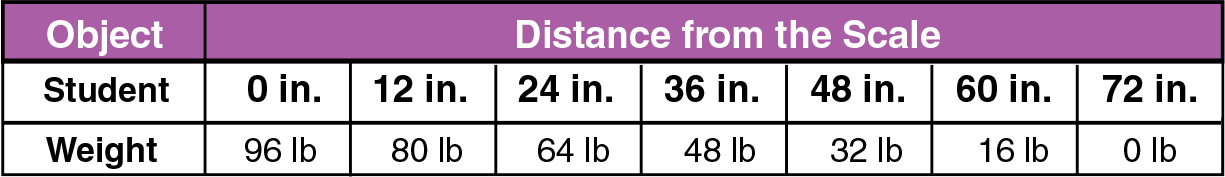After all of the weights have been recorded, have students plot the points (distance, weight) on a coordinate plane and draw a line through them.

The line should have a y-intercept at approximately the weight of the object and an x-intercept at approximately the length of the board. Have students write an equation of the line.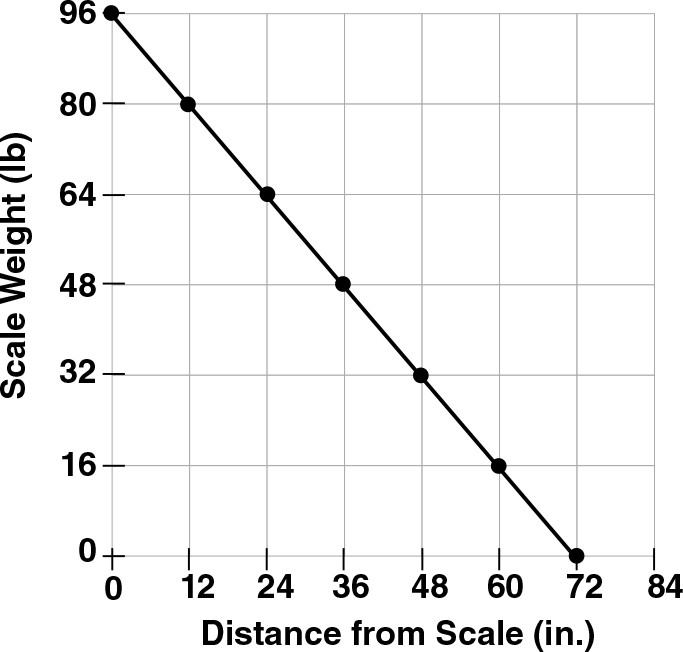#### Inquiry Strategies

1. Create Have students repeat the activity with several other objects. Have them explain the similarities and differences among the graphs. Possible response: The lines all have the same x-intercept, but the y-intercepts vary depending on the weight of the object. The lighter the object, the lesser the slope.
2. Analyze Tell students that a bag of potatoes placed on the scale weighs 24 pounds. Have them find the equation of the line that would represent the relationship between the distance from the scale and the weight shown on the scale. Ask, “What weight would be shown on the scale if the bag of potatoes was placed at the 36-inch mark?” Ask, “If the scale reads 17 pounds, how far is the bag of potatoes from the scale?”
y = 13x + 24; 12 lb; 21 in.

## Bar Graph Model

for Length-Area-Volume

### Construct a graphic display to show the relationships among the length of an edge and the surface area and volume of a cube.

1. Have students draw a cube with an edge length of 1 unit. Then have them construct two other cubes, one five times as large as the original cube and one ten times as large as the original cube. For each cube, find L (length of an edge), A (area of a face), SA (surface area), and V (volume).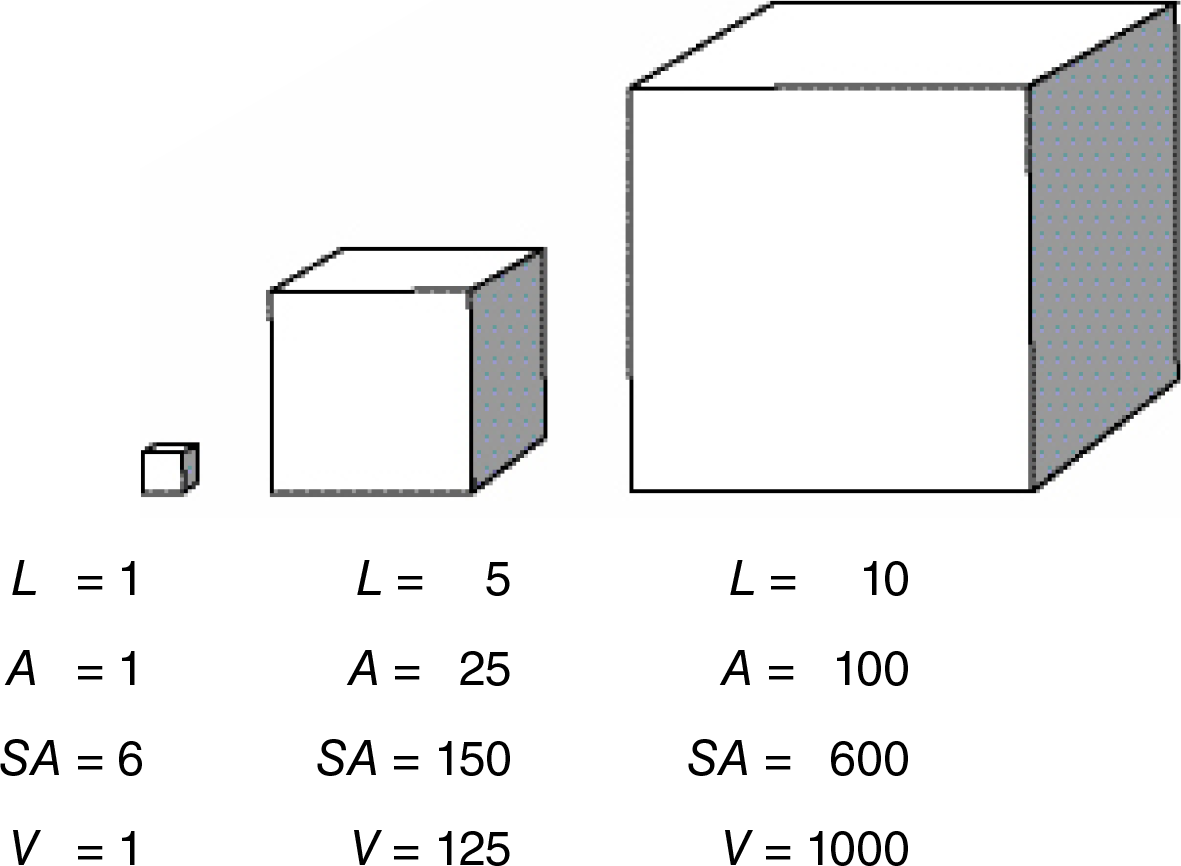1. Construct a graph that relates the length of an edge to the surface area and volume of a cube.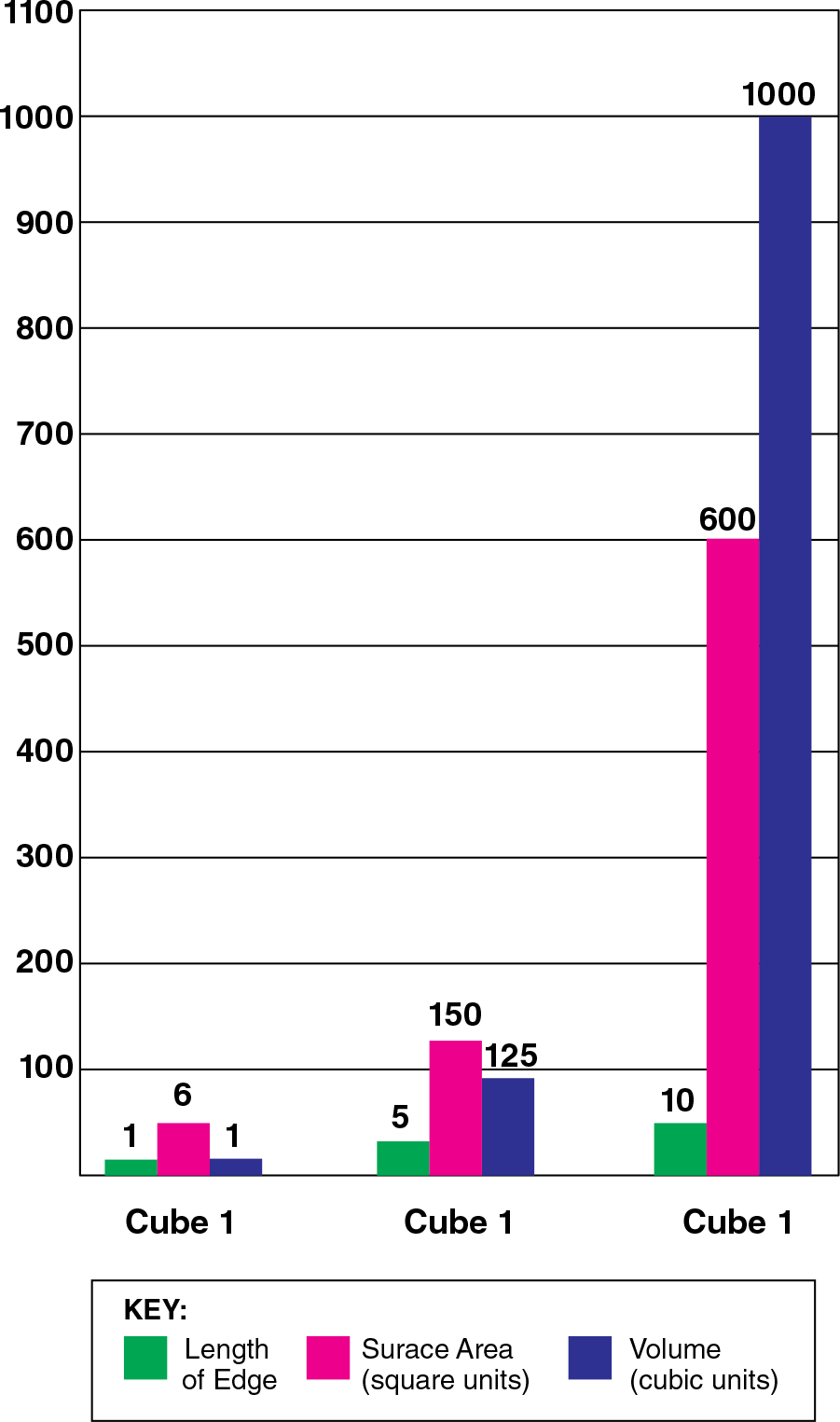#### Inquiry Strategies

1. Evaluate Ask, “If you make each edge of a cube 20 times longer, what will happen to the surface area, and what will happen to the volume? The surface area will be 400 times greater, and the volume will be 8000 times greater.
2. Analyze Ask, “As the length of an edge of a cube becomes greater, which increases faster: the surface area or the volume? Explain.” The volume increases faster. If the length of an edge of a cube is n, the surface area increases by a factor of n2, but the volume increases by a factor of n3.

## Working with Polynomials

### Different students relate to different models. The visuals below show how polynomials can be stacked and lined up for multiplication in the same way that students typically stack and line up multi-digit numbers.

Emphasize that this model is not the most efficient multiplication algorithm. Rather, it is intended to show how the partial products generated from multiplying polynomials are analogous to those generated from multiplying numbers.

Example with Numbers: Multiply 57 • 34.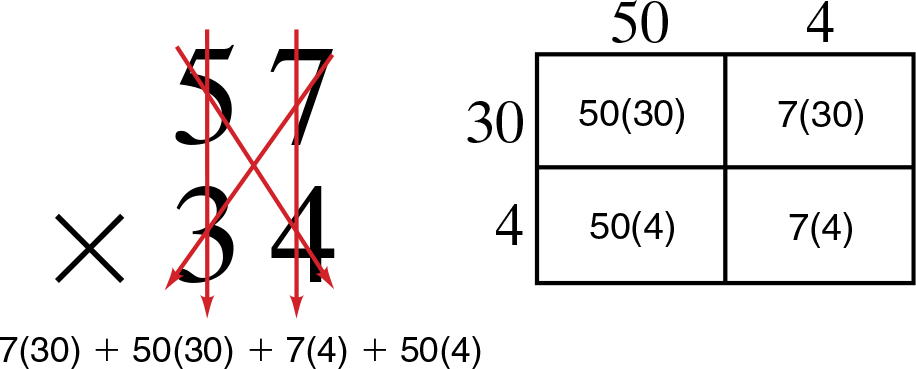Example with Polynomials: Multiply (3x – 6)(2x + 3)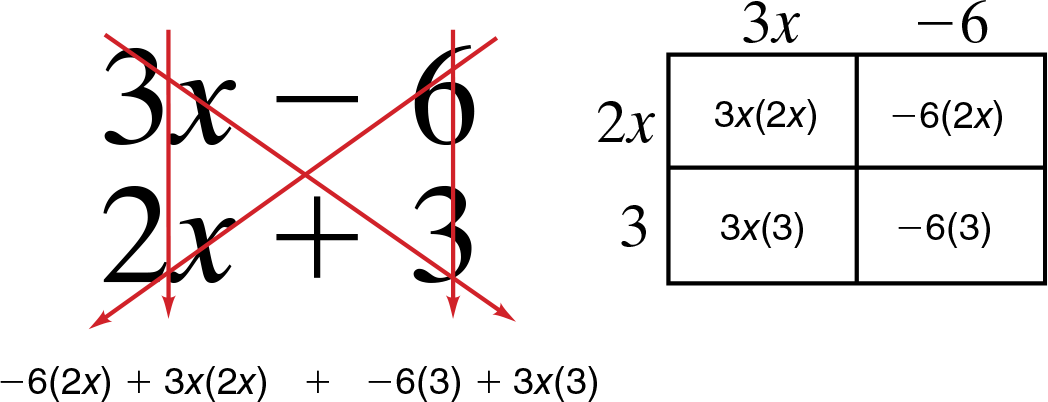#### Inquiry Strategies

1. Analyze On the board, write:Ask, “How would writing 57 • 34 in this way help you explain to a friend why multiplying binomials is like multiplying 2-digit numbers?” Responses may indicate that writing 57 as 5 tens + 7 makes it look more like a binomial, such as 3x – 6, so it is easier to see the connection between the two examples.
2. Apply Ask, “How many partial products will you get when you multiply a trinomial by a binomial?” Use the model to show why. 6; Students’ application of the model should show 6 arrows.

## Circle Model

for Finding the Total or Base

### If 40% of x is 12, what is x? Use a visual model to provide a picture of the solution.

• Draw a circle to represent the total, x.• Divide the circle into 40% sections, as follows: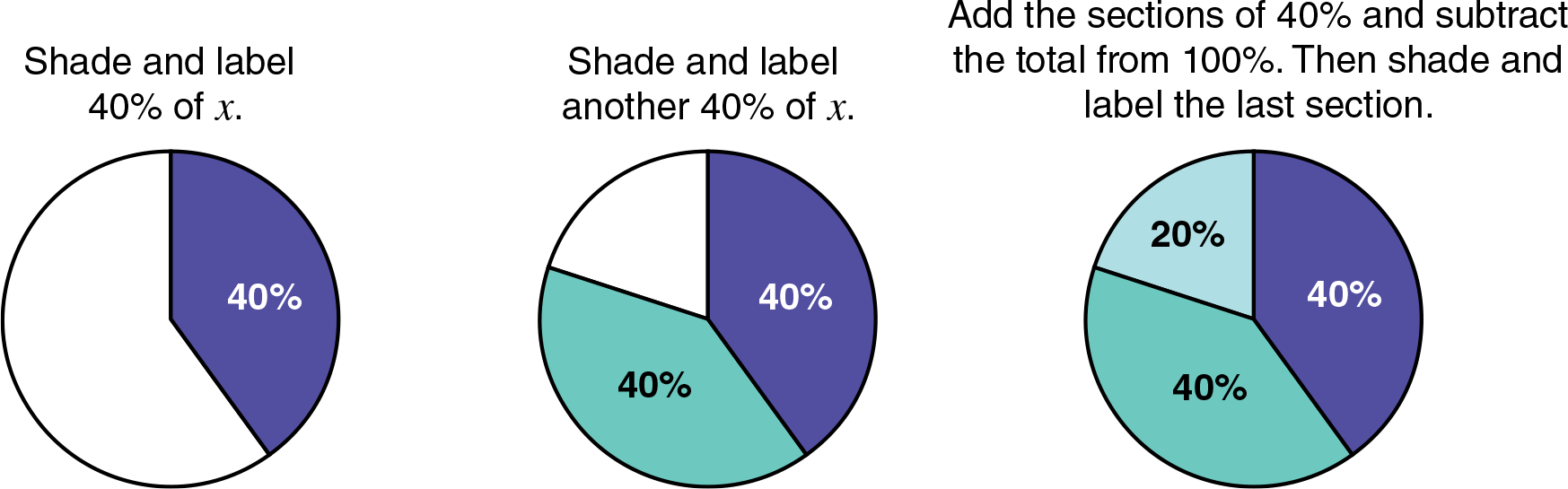• In each section, erase the percent and write the amount.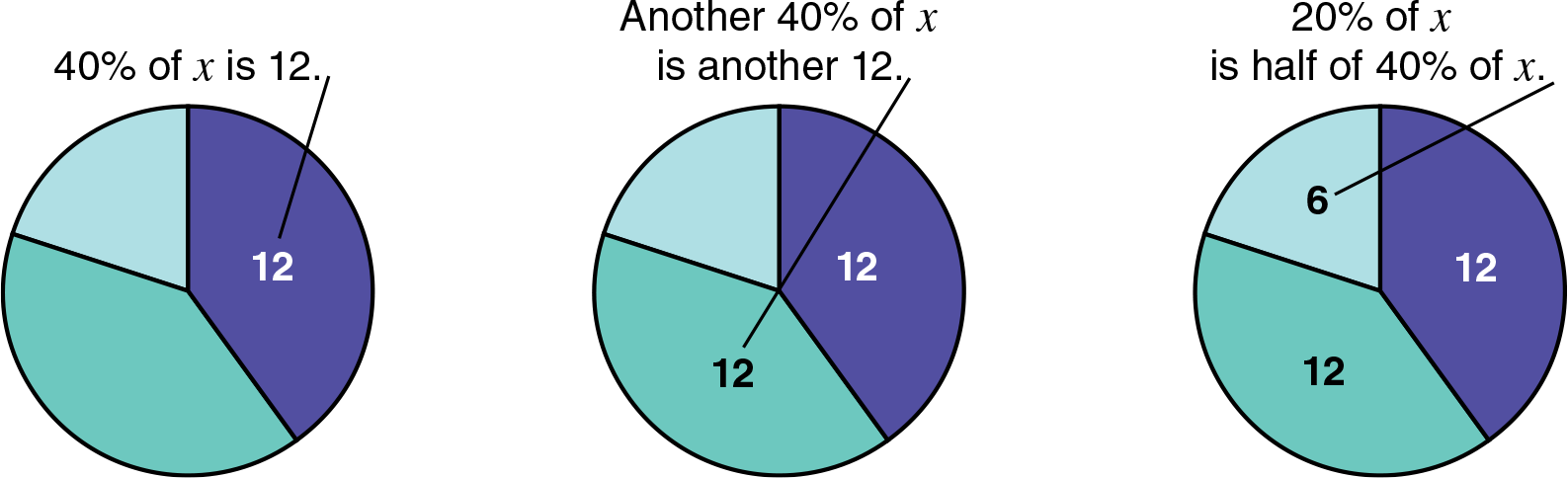So x = 12 + 12 + 6 = 30.

#### Inquiry Strategies

1. Analyze Write 10040 = 212 and 212 • 12 = 30 on the board. As you point to the equations, explain that someone solved the problem above by first dividing 100 by 40 and then multiplying the result by 12. Ask students how this computed solution relates to the model. Possible response: Like the computed solution, the circle model begins with finding how many 40% sections are in a whole and then finding the total if each 40% section represents 12.
2. Apply Have students use the model to find x, if 30% of x is 60. Students should show a circle divided into three 30% sections and one 10% section, which are then labeled 60, 60, 60, and 20. So x = 200.

## Geometric Models

for Proportions

### A geometric model for proportion takes advantage of students’ familiarity with analogies and other patterns that involve geometric figures.

• Place three physical models or drawings of different geometric figures in a standard proportion format. Have students place, draw, or describe the figure that belongs in the blank space.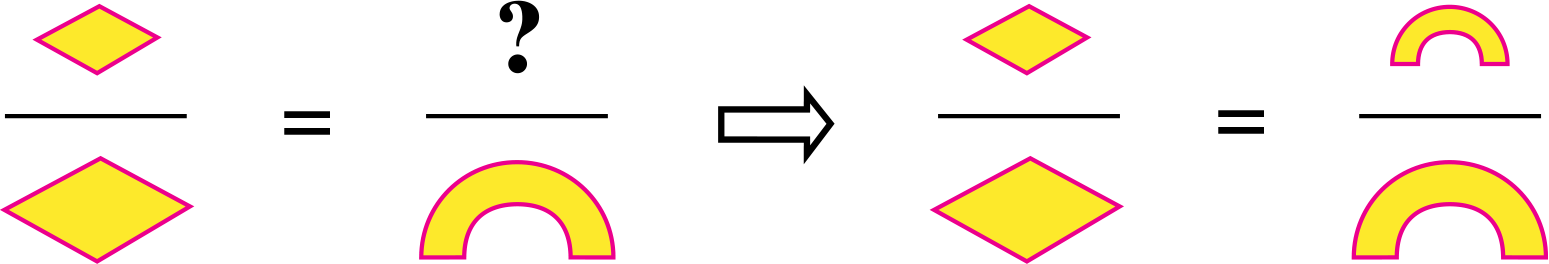• Apply the concept shown in the visual model above to trigonometric ratios. As you determine the other ratios in the following proportion, be ready to explain why the cosine of any given angle is constant.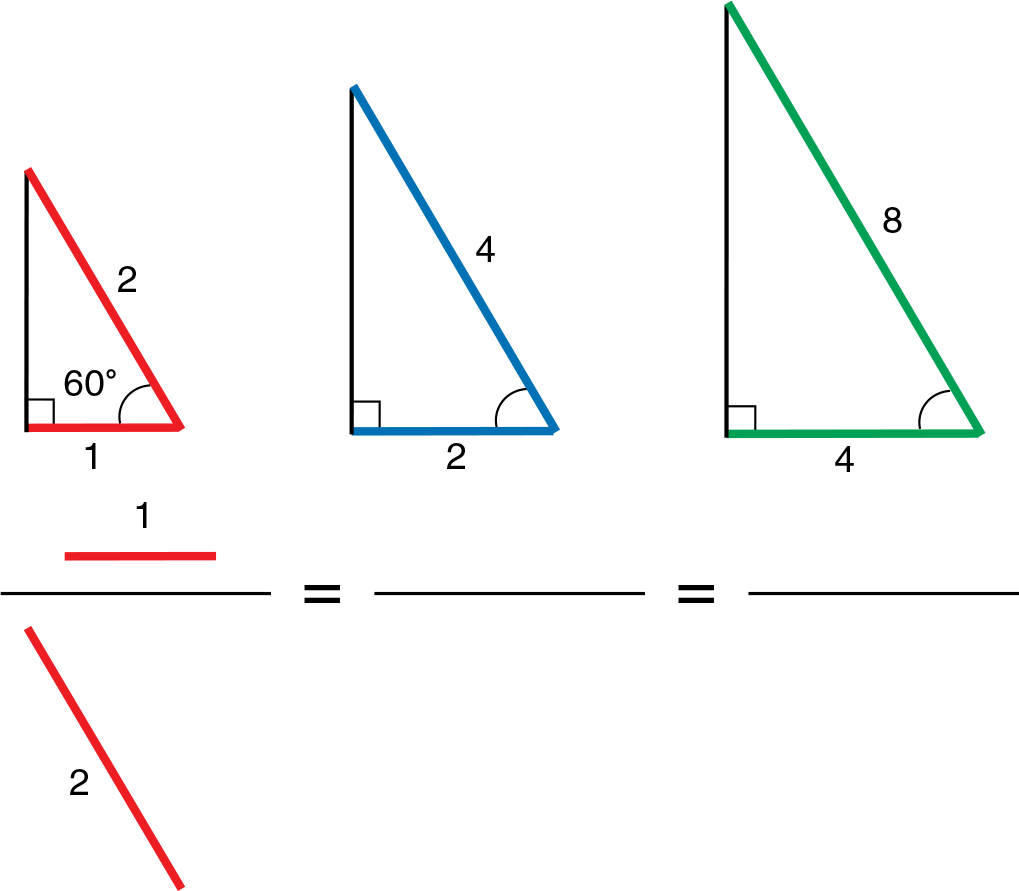#### Inquiry Strategies

1. Create Have students use the model above to illustrate the constancy of the tangent of a given angle. The drawing should be similar to the one above, but the numerator should be the opposite side (vertical leg) of the triangle and the denominator the adjacent side (horizontal leg).
2. Evaluate Ask, “When a photograph of a face is reduced proportionally, what ratios on the photograph stay the same?” Any ratio of two corresponding distances, such as length to width, the ratio of distance between eyes to width of smile, and so on.

## Area Model

for Pythagorean Theorem

### Cutting and rearranging the sections of an area model can help students see why the Pythagorean Theorem works for any right triangle, not just for special cases.

1. Draw any right triangle. From the sides, draw 3 squares to create an area model like the one shown at the right.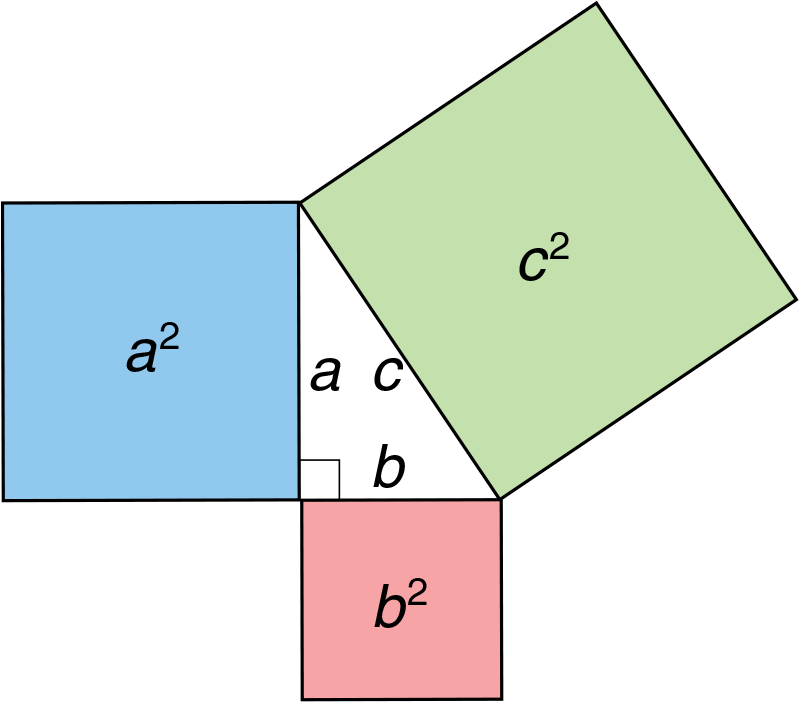1. Cut out the squares for a2 and b2.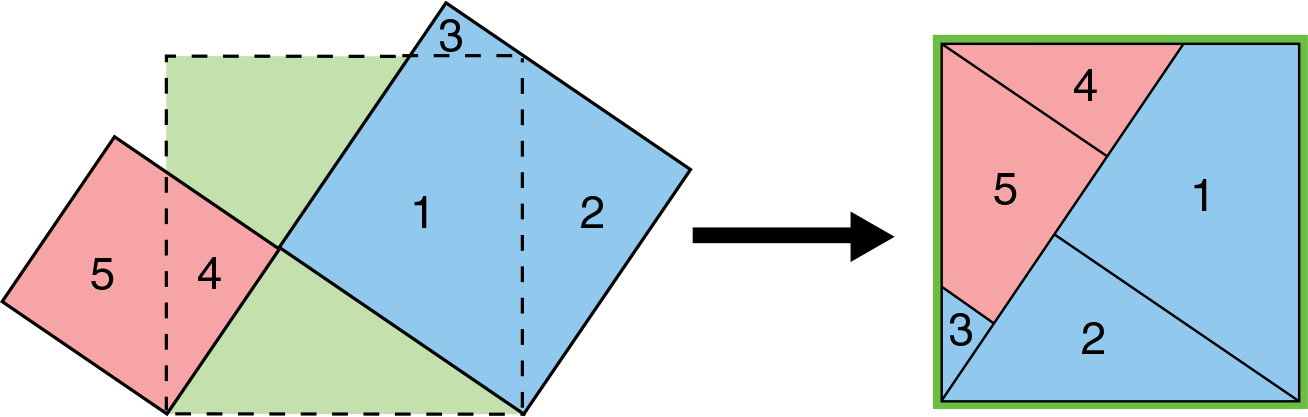1. Arrange the squares for a2 and b2 on top of the square for c2 as shown above. Cut a2 into 3 sections (1, 2, and 3) and cut b2 into 2 sections (4 and 5).
2. Rearrange the 5 sections to completely cover the square for c2.

#### Inquiry Strategies

1. Interpret Ask, “Does the model prove the Pythagorean Theorem is true? If not, what does it do?” Students should see that because cutting and rearranging sections is not exact, the model simply indicates the theorem is plausible and, more importantly, it applies to any side lengths, not just special ones.
2. Analyze When is the length of the hypotenuse of a right triangle irrational? The length is irrational whenever the sum of the squares of the lengths of the two legs is not a perfect square.

This method of cutting and arranging pieces appears on the website http://www.staff.hum.ku.dk/dbwagner/pythagoras/pythagoras. html and is credited to Professor Jöran Friberg, of the Department of Mathematics, Chalmers University of Technology, Gothenburg, Sweden.

## Coins or Counters

Models for Negative Numbers

### Models can help students understand numbers less than zero, but no model seems to work perfectly in all instances1. In the case of helping students differentiate between negative quantities and subtraction, coins or two–colored counters are well suited.

Use the two sides of a coin or counter to indicate 1 and its opposite (—1).Use the model to show the different meanings of “–” in a numerical expression.
Simplify — [—5 — (–2)]

Evaluate the expression step by step using the order of operations: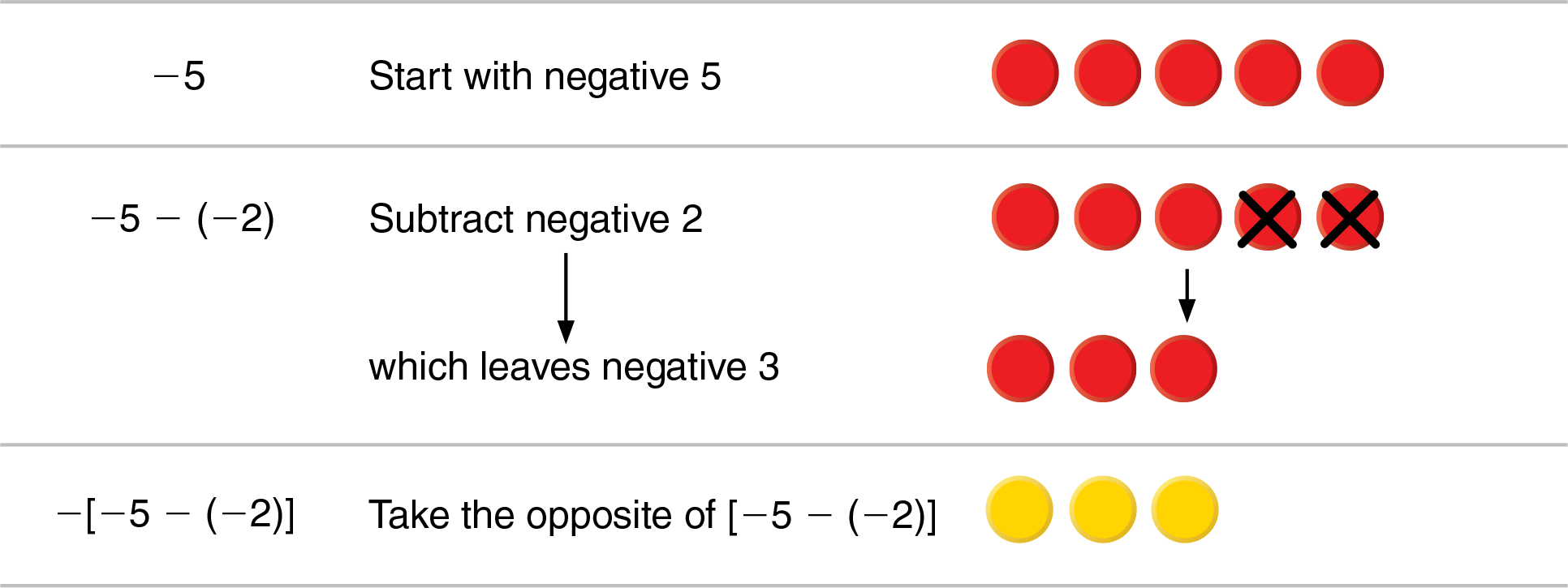So, –[–5 – (–2)] = 3.

#### Inquiry Strategies

1. Analyze Ask, “What are the two ways of using “—”? How are they different?” Students should recognize the distinction between subtracting, which means taking away or finding the difference and taking the opposite of, which means finding the additive inverse.
2. Apply On the board, write — (—1212). Ask, “What is the meaning of each sign?” Have students simplify the expression. The first means take the opposite of — (—1212). The second means take the opposite of 12. The third means subtract 12. The result is 1.

1 Fuson, Wearne, Hiebert, Murray, Human, Olivier, Carpenter, and Fennema, 1997; Hiebert, Carpenter, Fennema, Fuson, Wearne, Murray, Olivier, and Human, 1997 Cited in Adding it Up

## Balance Model

for Solving Equations

### With a balance model, a positive quantity is represented by a force (the weight of an object) pushing down on one of the pans. It makes sense to represent a negative quantity as the opposite, that is, a force pushing up on the pan.

Example: Solve 2x – 3 = 5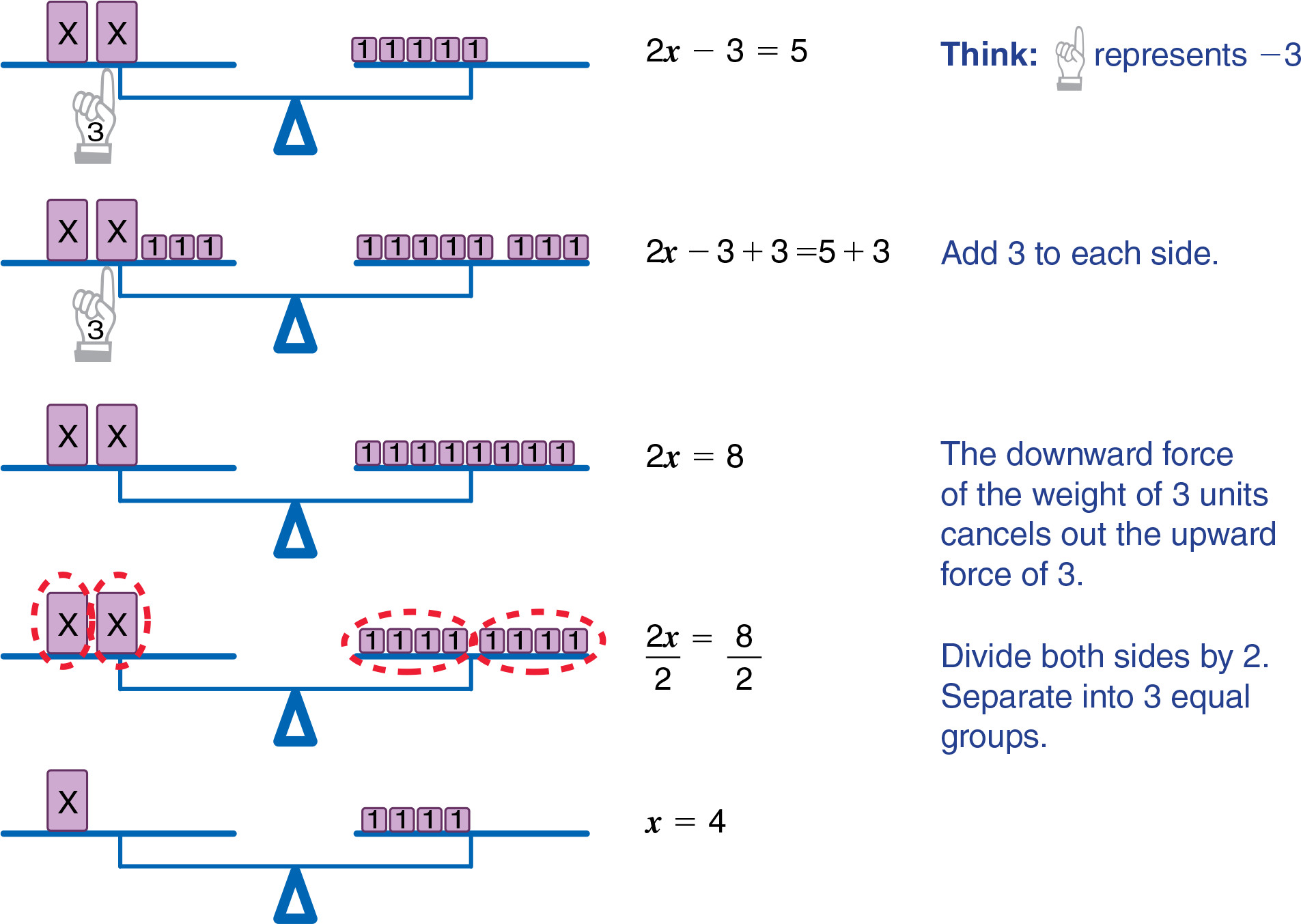#### Inquiry Strategies

1. Understand Ask, “Why does it make sense to show a negative number as a force pushing up?” A negative quantity is the opposite of the corresponding positive number; a force pushing up is the opposite of a force pushing down.
2. Apply On the board, write –3x + 4 = 19. Ask, “How would you use the balance model to solve this equation?” Left pan has 4 units and a force of 3x pulling up, represented by an object such as a balloon. Right pan has 19 units. Subtract 4 units from each side. Then, model division by separating the 15 units left on the right pan into 3 equal groups. When compared to the number of balloons, each x represents –5.

## Number Line Model

for Solving Inequalities

### Use a number line to show why the inequality sign must be reversed in some cases but not in others. Begin with a few instances of adding and subtracting the same quantity to two numbers. For example, start with 5 and 7.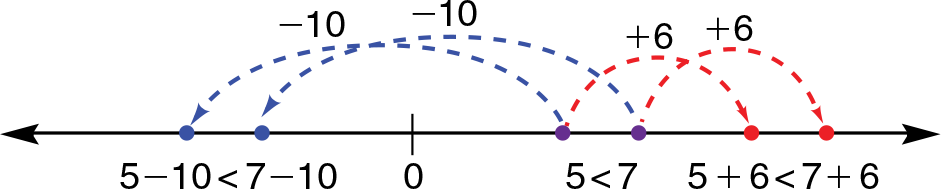Note that, in all cases, the resulting numbers compare in the same order. Then express this comparison in a general way using algebraic notation on the number line model.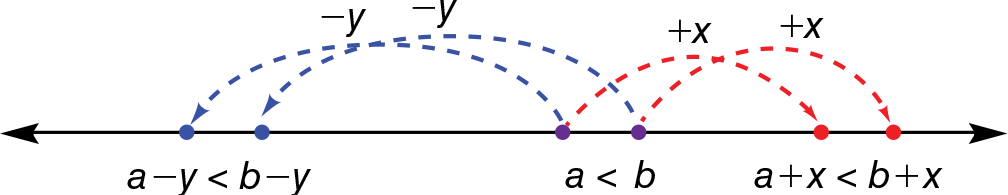Next, use the model to demonstrate that multiplying and dividing two numbers by the same positive amount also does not change the way the results compare.

Then use the model with multiplication and division by negative quantities so students can see that the order does change. For example, start with 6 and 10, and divide both by —2.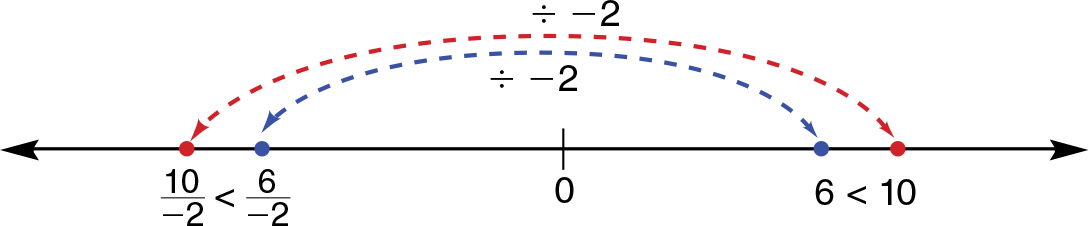Then demonstrate the more general situation by comparing variables a and b with an inequality sign, and then multiplying both sides by —1.#### Inquiry Strategies

1. Understand Ask, “How would you demonstrate to a friend that the inequality sign is reversed when you multiply or divide both sides of an inequality by a negative quantity?” Students should use a number line drawing to show that multiplying or dividing by a negative amount flips the numbers to the opposite side of the number line and changes the comparison.
2. Apply On the board, write —23x > –12. Ask, “If you multiply both sides by 32, does the inequality sign reverse?” What about, if in the next step, you multiply both sides by –1?” no; yes